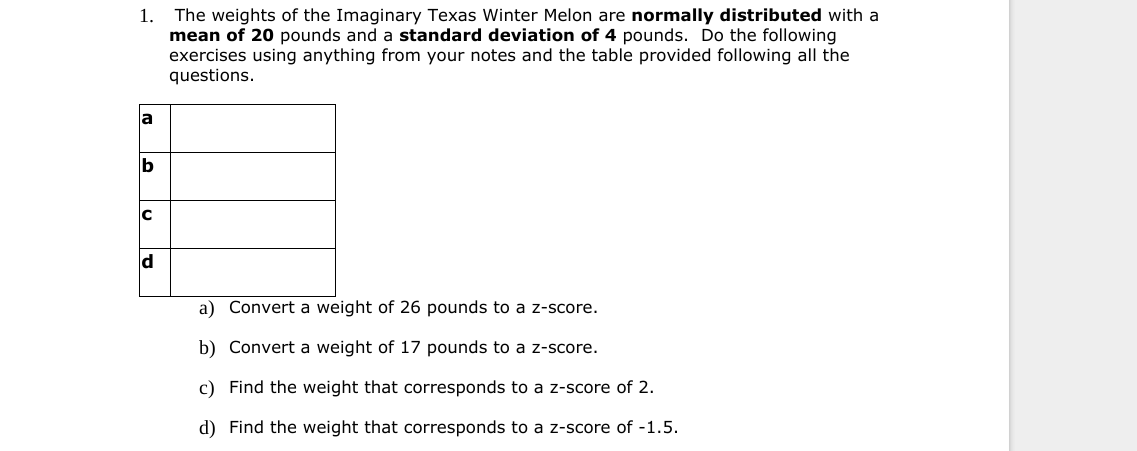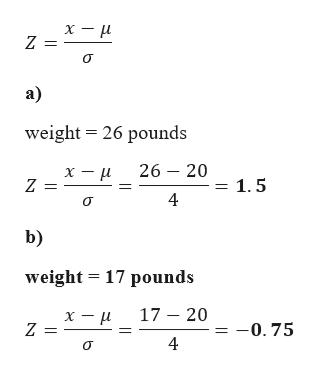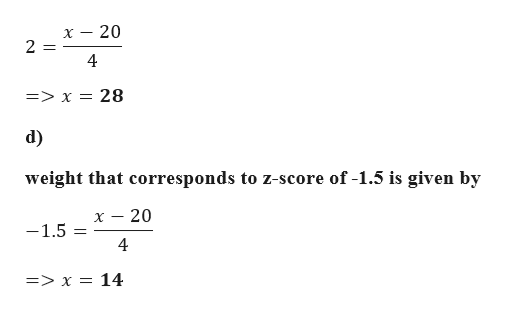# The weights of the Imaginary Texas Winter Melon are normally distributed with amean of 20 pounds and a standard deviation of 4 pounds. Do the followingexercises using anything from your notes and the table provided following all thequestions1.aCa) Convert a weight of 26 pounds to a z-score.b) Convert a weight of 17 pounds to a z-score.c) Find the weight that corresponds to a z-score of 2.d) Find the weight that corresponds to a z-score of -1.5.

Question
69 viewshelp_outlineImage TranscriptioncloseThe weights of the Imaginary Texas Winter Melon are normally distributed with a mean of 20 pounds and a standard deviation of 4 pounds. Do the following exercises using anything from your notes and the table provided following all the questions 1. a C a) Convert a weight of 26 pounds to a z-score. b) Convert a weight of 17 pounds to a z-score. c) Find the weight that corresponds to a z-score of 2. d) Find the weight that corresponds to a z-score of -1.5. fullscreen
check_circle

Step 1

Given data

Mean = 20

Standard Deviation = 4

z-score is given by the following formulahelp_outlineImage Transcriptioncloseх — и Z = а) weight 26 pounds 26 20 = 1.5 х — и 4 b) weight 17 pounds 17 20 х — и -0. 75 4 fullscreen
Step 2

c)

weight that corresponds to z-score of 2 is g...help_outlineImage Transcriptionclose- 20 4 => x = 28 d) weight that corresponds to z-score of -1.5 is given by - 20 -1.5 4 => x = 14 fullscreen

### Want to see the full answer?

See Solution

#### Want to see this answer and more?

Solutions are written by subject experts who are available 24/7. Questions are typically answered within 1 hour.*

See Solution
*Response times may vary by subject and question.
Tagged in

### Other